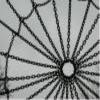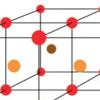# Resources tagged with: Generalising

Filter by: Content type:
Age range:
Challenge level:

### There are 149 results

Broad Topics > Thinking Mathematically > Generalising### Shear Magic

##### Age 11 to 14Challenge Level

Explore the area of families of parallelograms and triangles. Can you find rules to work out the areas?### Christmas Chocolates

##### Age 11 to 14Challenge Level

How could Penny, Tom and Matthew work out how many chocolates there are in different sized boxes?### Sliding Puzzle

##### Age 11 to 16Challenge Level

The aim of the game is to slide the green square from the top right hand corner to the bottom left hand corner in the least number of moves.### Cubes Within Cubes Revisited

##### Age 11 to 14Challenge Level

Imagine starting with one yellow cube and covering it all over with a single layer of red cubes, and then covering that cube with a layer of blue cubes. How many red and blue cubes would you need?### Hidden Rectangles

##### Age 11 to 14Challenge Level

Rectangles are considered different if they vary in size or have different locations. How many different rectangles can be drawn on a chessboard?### Picturing Triangular Numbers

##### Age 11 to 14Challenge Level

Triangular numbers can be represented by a triangular array of squares. What do you notice about the sum of identical triangle numbers?### Picturing Square Numbers

##### Age 11 to 14Challenge Level

Square numbers can be represented as the sum of consecutive odd numbers. What is the sum of 1 + 3 + ..... + 149 + 151 + 153?### Games Related to Nim

##### Age 5 to 16

This article for teachers describes several games, found on the site, all of which have a related structure that can be used to develop the skills of strategic planning.### Tourism

##### Age 11 to 14Challenge Level

If you can copy a network without lifting your pen off the paper and without drawing any line twice, then it is traversable. Decide which of these diagrams are traversable.### Window Frames

##### Age 5 to 14Challenge Level

This task encourages you to investigate the number of edging pieces and panes in different sized windows.### Dotty Triangles

##### Age 11 to 14Challenge Level

Imagine an infinitely large sheet of square dotty paper on which you can draw triangles of any size you wish (providing each vertex is on a dot). What areas is it/is it not possible to draw?### Number Pyramids

##### Age 11 to 14Challenge Level

Try entering different sets of numbers in the number pyramids. How does the total at the top change?### Got It

##### Age 7 to 14Challenge Level

A game for two people, or play online. Given a target number, say 23, and a range of numbers to choose from, say 1-4, players take it in turns to add to the running total to hit their target.### Route to Infinity

##### Age 11 to 14Challenge Level

Can you describe this route to infinity? Where will the arrows take you next?### Go Forth and Generalise

##### Age 11 to 14

Spotting patterns can be an important first step - explaining why it is appropriate to generalise is the next step, and often the most interesting and important.### Maths Trails

##### Age 7 to 14

The NRICH team are always looking for new ways to engage teachers and pupils in problem solving. Here we explain the thinking behind maths trails.### Dotty Circle

##### Age 7 to 11Challenge Level

Watch this film carefully. Can you find a general rule for explaining when the dot will be this same distance from the horizontal axis?### Frogs

##### Age 11 to 14Challenge Level

How many moves does it take to swap over some red and blue frogs? Do you have a method?### Coordinate Patterns

##### Age 11 to 14Challenge Level

Charlie and Alison have been drawing patterns on coordinate grids. Can you picture where the patterns lead?### Tilted Squares

##### Age 11 to 14Challenge Level

It's easy to work out the areas of most squares that we meet, but what if they were tilted?### More Twisting and Turning

##### Age 11 to 16Challenge Level

It would be nice to have a strategy for disentangling any tangled ropes...### Partitioning Revisited

##### Age 11 to 14Challenge Level

We can show that (x + 1)² = x² + 2x + 1 by considering the area of an (x + 1) by (x + 1) square. Show in a similar way that (x + 2)² = x² + 4x + 4### Cuboid Challenge

##### Age 11 to 16Challenge Level

What's the largest volume of box you can make from a square of paper?### More Number Pyramids

##### Age 11 to 14Challenge Level

When number pyramids have a sequence on the bottom layer, some interesting patterns emerge...### Is There a Theorem?

##### Age 11 to 14Challenge Level

Draw a square. A second square of the same size slides around the first always maintaining contact and keeping the same orientation. How far does the dot travel?##### Age 11 to 14Challenge Level

A little bit of algebra explains this 'magic'. Ask a friend to pick 3 consecutive numbers and to tell you a multiple of 3. Then ask them to add the four numbers and multiply by 67, and to tell you. . . .##### Age 11 to 16

Dave Hewitt suggests that there might be more to mathematics than looking at numerical results, finding patterns and generalising.### One, Three, Five, Seven

##### Age 11 to 16Challenge Level

A game for 2 players. Set out 16 counters in rows of 1,3,5 and 7. Players take turns to remove any number of counters from a row. The player left with the last counter looses.### Taking Steps

##### Age 7 to 11Challenge Level

In each of the pictures the invitation is for you to: Count what you see. Identify how you think the pattern would continue.### Squares, Squares and More Squares

##### Age 11 to 14Challenge Level

Can you dissect a square into: 4, 7, 10, 13... other squares? 6, 9, 12, 15... other squares? 8, 11, 14... other squares?### Squares in Rectangles

##### Age 11 to 14Challenge Level

A 2 by 3 rectangle contains 8 squares and a 3 by 4 rectangle contains 20 squares. What size rectangle(s) contain(s) exactly 100 squares? Can you find them all?### Nim-7 for Two

##### Age 5 to 14Challenge Level

Nim-7 game for an adult and child. Who will be the one to take the last counter?### Winning Lines

##### Age 7 to 16

An article for teachers and pupils that encourages you to look at the mathematical properties of similar games.### Egyptian Fractions

##### Age 11 to 14Challenge Level

The Egyptians expressed all fractions as the sum of different unit fractions. Here is a chance to explore how they could have written different fractions.### Finding 3D Stacks

##### Age 7 to 11Challenge Level

Can you find a way of counting the spheres in these arrangements?##### Age 11 to 14Challenge Level

List any 3 numbers. It is always possible to find a subset of adjacent numbers that add up to a multiple of 3. Can you explain why and prove it?### Enclosing Squares

##### Age 11 to 14Challenge Level

Can you find sets of sloping lines that enclose a square?### Sum Equals Product

##### Age 11 to 14Challenge Level

The sum of the numbers 4 and 1 [1/3] is the same as the product of 4 and 1 [1/3]; that is to say 4 + 1 [1/3] = 4 ï¿½ 1 [1/3]. What other numbers have the sum equal to the product and can this be. . . .### Special Sums and Products

##### Age 11 to 14Challenge Level

Find some examples of pairs of numbers such that their sum is a factor of their product. eg. 4 + 12 = 16 and 4 × 12 = 48 and 16 is a factor of 48.### Triangle Pin-down

##### Age 7 to 11Challenge Level

Use the interactivity to investigate what kinds of triangles can be drawn on peg boards with different numbers of pegs.### Always, Sometimes or Never? Shape

##### Age 7 to 11Challenge Level

Are these statements always true, sometimes true or never true?### Circles, Circles

##### Age 5 to 11Challenge Level

Here are some arrangements of circles. How many circles would I need to make the next size up for each? Can you create your own arrangement and investigate the number of circles it needs?### Magic Letters

##### Age 11 to 14Challenge Level

Charlie has made a Magic V. Can you use his example to make some more? And how about Magic Ls, Ns and Ws?### Seven Squares - Group-worthy Task

##### Age 11 to 14Challenge Level

Choose a couple of the sequences. Try to picture how to make the next, and the next, and the next... Can you describe your reasoning?### Tower of Hanoi

##### Age 11 to 14Challenge Level

The Tower of Hanoi is an ancient mathematical challenge. Working on the building blocks may help you to explain the patterns you notice.### Dicing with Numbers

##### Age 11 to 14Challenge Level

In how many ways can you arrange three dice side by side on a surface so that the sum of the numbers on each of the four faces (top, bottom, front and back) is equal?### One O Five

##### Age 11 to 14Challenge Level

You can work out the number someone else is thinking of as follows. Ask a friend to think of any natural number less than 100. Then ask them to tell you the remainders when this number is divided by. . . .### Chocolate Maths

##### Age 11 to 14Challenge Level

Pick the number of times a week that you eat chocolate. This number must be more than one but less than ten. Multiply this number by 2. Add 5 (for Sunday). Multiply by 50... Can you explain why it. . . .### Overlap

##### Age 11 to 14Challenge Level

A red square and a blue square overlap so that the corner of the red square rests on the centre of the blue square. Show that, whatever the orientation of the red square, it covers a quarter of the. . . .### 2001 Spatial Oddity

##### Age 11 to 14Challenge Level

With one cut a piece of card 16 cm by 9 cm can be made into two pieces which can be rearranged to form a square 12 cm by 12 cm. Explain how this can be done.